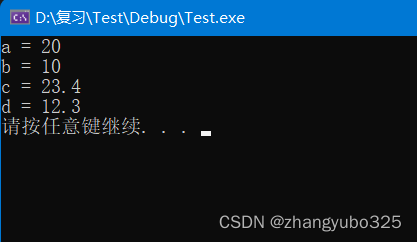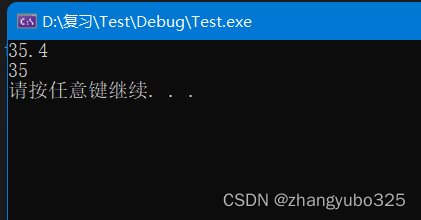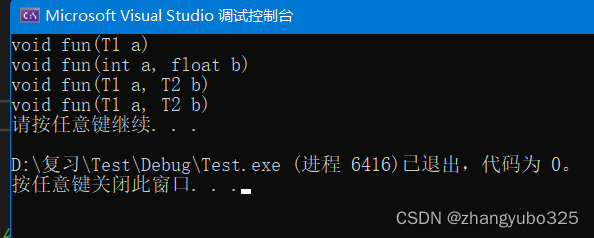# 泛型编程与函数模板

C++的一种编程思想称为泛型编程，主要利用的技术就是模板

## 函数模板的用法

1.template < typename T>
2.函数的声明或定义

template：声明创建模板；
typename：表明其后面的符号是一种数据类型，可以用class代替；
T：通用的数据类型，名称可以替换，通常为大写字母。

template // 模板参数列表 ———— 参数类型
void Swap(T& rx, T& ry) { // 函数参数列表 ———— 参数对象
T tmp = rx;
rx = ry;
ry = tmp;
}

template<typename T1, typename T2, typename T3>

## 注意事项及引入代码测试

• 函数模板不是一个函数，因为它不是具体要调用的某一个函数，而是一个模板；

• 自动类型推导，必须推导出一致的数据类型T才可以使用；模板必须要确定出T的数据类型才可以使用；

• 可以通过空模板实参列表< >限定编译器只匹配函数模板

• 我们在用 template< > 定义模板的时候，尖括号里的 typename 其实还可以写成 class；

template // 使用class充当typename
void Swap(T& rx, T& ry) {
T tmp = rx;
rx = ry;
ry = tmp;
}

## 完整代码测试

#include <iostream>

using namespace std;

template <typename T>
void Swap(T& a, T& b)
{
T tmp = a;
a = b;
b = tmp;
}

void main()
{
int a = 10;
int b = 20;
Swap(a, b);	//自动推到调用
//Swap<int>(a, b);//显示指定调用
cout << "a = " << a << endl;
cout << "b = " << b << endl;

float c = 12.3;
float d = 23.4;

//Swap(c, d); //自动推到调用
Swap<float>(c, d); //显示指定调用
cout << "c = " << c << endl;
cout << "d = " << d << endl;
system("pause");
}### 1.深入理解函数模板：

• 对于函数模板中使用的类型不同，编译器会产生不同的函数
• 编译器会对函数模板进行两次编译
• 第一次是对函数模板本身进行编译，包括语法检查等
• 第二次是对参数替换后的代码进行编译，这就相当于编译普通函数一样，进行类型规则检查等。

• 函数模板是不允许隐式类型转换的，调用时类型必须严格匹配；

• 函数模板还可以定义任意多个不同的类型参数，但是对于多参数函数模板；

• 编译器是无法自动推导返回值类型的，可以从左向右部分指定类型参数。

#include <iostream>

using namespace std;

template <typename T1, typename T2, typename T3>
{
T1 ret;
ret = static_cast<T1>(a + b);
return ret;
}

void main()
{
int c = 12;
float d = 23.4;
//cout << add(c, d) << endl;	//error，无法自动推导函数返回值
cout << add<float>(c, d) << endl;	//**返回值在第一个类型参数中指定**
cout << add<int, int, float>(c, d) << endl;
system("pause");
}### 2.函数模板的重载

• C++编译器优先考虑普通函数；

• 如果函数模板可以产生一个更好的匹配，那么就选择函数模板；

#include <iostream>

using namespace std;

template <typename T>
void fun(T a)
{
cout << "void fun(T1 a)" << endl;
}

template <typename T1, typename T2>
void fun(T1 a, T2 b)
{
cout << "void fun(T1 a, T2 b)" << endl;
}

void fun(int a, float b)
{
cout << "void fun(int a, float b)" << endl;
}

void main()
{
int a = 0;
float b = 0.0;
fun(a);
fun(a, b);	//普通函数void fun(int a, float b)已经能完美匹配，于是调用普通函数
fun(b, a);	//这个调用，函数模板有更好的匹配，于是调用函数模板
fun<>(a, b);	//限定只使用函数模板
system("pause");
}## 总结

• 函数模板是泛型编程在C++中的应用方式之一
• 函数模板能够根据实参对参数类型进行推导
• 函数模板支持显示的指定参数类型
• 函数模板是C++中重要的代码复用方式
• 函数模板通过具体类型产生不同的函数
• 函数模板可以定义任意多个不同的类型参数
• 函数模板中的返回值类型必须显示指定
• 函数模板可以像普通函数一样重载
10-2787
02-25542
11-286259
11-252353
10-222287
04-156886
11-09391
09-045250
10-15747
08-31
10-16
05-02131
07-09326
08-181万+
07-279125

### “相关推荐”对你有帮助么？

•非常没帮助
•没帮助
•一般
•有帮助
•非常有帮助被折叠的  条评论 为什么被折叠?到【灌水乐园】发言zhangyubo325

¥2 ¥4 ¥6 ¥10 ¥20余额支付 (余额：-- )扫码支付获取中扫码支付点击重新获取扫码支付1.余额是钱包充值的虚拟货币，按照1:1的比例进行支付金额的抵扣。
2.余额无法直接购买下载，可以购买VIP、C币套餐、付费专栏及课程。余额充值